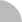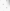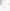# 臺灣博碩士論文加值系統

(3.238.135.174) 您好！臺灣時間：2021/08/05 07:42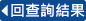:::

### 詳目顯示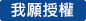: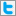Twitter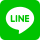•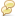被引用:0
•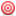點閱:52
•評分: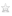•下載:0
•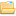書目收藏:0
 本論文主要在探討由內往外的序列或連續檢定程序來檢測出雙參數指數樣本中 k 個較大的離群值。我們總共考慮六個檢定統計量，其中一個統計量是根據樣本中可能不被懷疑為離群值的觀測值，計算其中最大觀測值和平均數之間的差與其相對樣本全距之比率；其他五個檢定統計量則是分別在 Basu (1965)、Balasooriya and Gadag (1994)、Zerbet and Nikulin (2003) 和 Kumar (2013b) 的論文中所討論過的區塊檢定程序所使用的統計量。利用Huffer (1988)的遞迴關係式和 Lin and Balakrishnan (2009) 的演算法，我們分別找出以上六個檢定統計量在 k = 2 和 3 時，雙參數指數中 k 個較大的離群值的連續檢定程序之聯合虛無分配的臨界值。最後，再根據蒙地卡羅模擬的方法來比較這六個檢定統計量的檢定力和錯誤判定機率。
 The inside-out sequential procedures for testing up to k upper outliers in a two-parameter exponential sample are investigated. Six test statistics, one based on the ratio of the difference of largest observation and the sample mean which are unsuspected to be outliers to the range of these observations, and others used for block test procedures discussed in Basu (1965), Balasooriya and Gadag (1994), Zerbet and Nikulin (2003), and Kumar (2013b), are considered. Utilizing the recursion of Huffer (1988) and algorithm of Lin and Balakrishnan (2009), the critical values of the joint null distributions of these test statistics for sequential testing discordancy of k upper outliers in two-parameter exponential samples on the important cases k = 2 and 3 are obtained. Powers of tests based on these statistics are compared through a Monte Carlo study.
 Contents1 Introduction 12 The Distribution of Test Statistics 43 Illustrative Examples 64 Performance Comparison 105 Concluding Remarks 13References 13Appendix 16List of TablesTable 1 Exact critical values of test statistics (3) to (8) for j = 1, 2, and the associated value of β when α = 0.05. . . . . . . . . . . . . . . . . . . . . . . . . . . . . . . 7Table 2 Exact critical values of test statistics (3) to (8) for j = 1, 2, 3, and the associated value of β when α = 0.05. . . . . . . . . . . . . . . . . . . . . . . . . 8Table 3 Exact critical values of test statistics and the corresponding observed statistics for j = 1, . . . , k when k ≤ 4 in Example 1. . . . . . . . . . . . . . . . . . . 9Table 4 Exact critical values of test statistics and the corresponding observed statistics for j = 1, . . . , k when k ≤ 4 in Example 2. . . . . . . . . . . . . . . . . . . 11List of FiguresFigure 1 Powers and error probabilities of testing procedures for n = 10 when k = 2. 20Figure 2 Powers and error probabilities of testing procedures for n = 15 when k = 2. 21Figure 3 Powers and error probabilities of testing procedures for n = 20 when k = 2. 22Figure 4 Powers and error probabilities of testing procedures for n = 15 when k = 3. 23Figure 5 Powers and error probabilities of testing procedures for n = 30 when k = 3. 24Figure 6 Powers and error probabilities of testing procedures for n = 50 when k = 3. 25Figure 7 Powers and error probabilities of testing procedures for n = 100 when k = 3. 26
 Arnold, B. C., Balakrishnan, N. and Nagaraja, H. N. (1992). A First Course in Order Statistics. John Wiley & Sons, New York.Balasooriya, U. and Gadag, V. (1994). Tests for upper outliers in the two-parameter exponential distribution. Journal of Statistical Computation and Simulation 50, 249–259.Barnett, V. and Lewis, T. (1994). Outliers in Statistical Data. Third Edition, John Wiley & Sons, Chichester, England.Basu, A. P. (1965). On some tests of hypotheses relating to the exponential distribution when some outliers are present. Journal of the American Statistical Association 60, 548–559.Chen, G. and Balakrishnan, N. (1995). A general purpose approximate goodness-of-fit test. Journal of Quality Technology 27, 154–161.Chikkagoudar, M. S. and Kunchur, S. H. (1983). Distributions of test statistics for multiple outliers in exponential samples. Communications in Statistics – Theory and Methods 12, 2127–2142.Chikkagoudar, M. S. and Kunchur, S. H. (1987). Comparison of many outlier procedures for exponential samples. Communications in Statistics – Theory and Methods 16, 627–645.Epstein, B. (1960). Tests for the validity of the assumption that the underlying distribution of life is exponential: Part II. Technometrics 2, 167–183.Fieller, N. R. J. (1976). Some problems related to the rejection of outlying observations. Ph.D. Thesis, University of Hull.Huffer, F. (1988). Divided differences and the joint distribution of linear combinations of spacings. Journal of Applied Probability 25, 346–354.Huffer, F. W. and Lin, C. T. (2001). Computing the joint distribution of general linear combinations of spacings or exponential variates. Statistica Sinica 11, 1141–1157.Huffer, F. W. and Lin, C. T. (2006). Linear combinations of spacings. In Encyclopedia of Statistical Sciences, Volume 12, Second Edition (Eds., S. Kotz, N. Balakrishnan, C. B.Read and B. Vidakovic), pp. 7866–7875, John Wiley & Sons, Hoboken, New Jersey.Kimber, A. C. (1982). Tests for many outliers in an exponential sample. Applied Statistics 31, 263–271.Kimber, A. C. and Stevens, H. J. (1981). The null distribution of a test for two upper outliers in an exponential sample. Applied Statistics 30, 153–157.Kumar, N. (2013a). Test for multiple upper outliers in an exponential sample irrespective of origin. Statistics 47, 184–190.Kumar, N. (2013b). Test for suspected observations in an exponential sample with unknown origin, unpublished manuscript.Kumar, N. and Lalitha, S. (2012). Testing for upper outliers in gamma sample. Communications in Statistics – Theory and Methods 41, 820–828.Lewis, T. and Fieller, N. R. J. (1979). A recursive algorithm for null distribution for outliers: I. Gamma samples. Technometrics 21, 371–376.Lin, C. T. and Balakrishnan, N. (2009). Exact computation of the null distribution of a test for multiple outliers in an exponential sample. Computational Statistics & Data Analysis 53, 3281–3290.Lin, C. T. and Balakrishnan, N. (2013). Tests for multiple outliers from an exponential population. Communications in Statistics – Simulation and Computation. http://DOI:10.1080/03610918.2012.714030.Proschan, F. (1963). Theoretical explanation of observed decreasing failure rate. Technometrics 5, 375–383.Rosner, B. (1975). On the detection of many outliers. Technometrics 17, 221–227.Tietjen, G. L. and Moore, R. H. (1972). Some Grubbs-type statistics for the detection of several outliers. Technometrics 14, 583–597.Zerbet, A. and Nikulin, M. (2003). A new statistic for detecting outliers in exponential case. Communications in Statistics – Theory and Methods 32, 573–583.Zhang, J. (1998). Tests for multiple upper or lower outliers in an exponential sample. Journal of Applied Statistics 25, 245–255.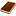國圖紙本論文推文當script無法執行時可按︰推文 網路書籤當script無法執行時可按︰網路書籤 推薦當script無法執行時可按︰推薦 評分當script無法執行時可按︰評分 引用網址當script無法執行時可按︰引用網址 轉寄當script無法執行時可按︰轉寄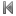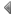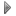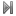top
 1 運用穩健之多變量管制圖於異常點偵測之研究

 無相關期刊

 1 處理離群值之多目標規劃模糊迴歸法 2 韋伯型I混合設限資料之加速壽命試驗的推論 3 連續時間隨機波動模型的適合度檢定 4 多類別名目型資料的邏輯斯迴歸模型之適合度檢定統計量研究 5 Person-fit偵測作假之效用- 非參數試題反應理論的模擬與應用 6 應用模糊理論於具離群值之時間序列參數的估計及預測 7 基於影像不變特徵及區域離群值檢測之重複區域偵測 8 進階回交族群之數量性狀基因座定位門檻值研究 9 Semiparametric Tests for Nonstationary Data 10 多類別時間序列模型之適合度檢定統計量研究 11 檢測向量自我迴歸模型下概似比的 p-value 估計 12 在自我相似過程中的嵌入分支過程之研究 13 離群值比例之基因分析 14 利用離群值偵測技術於無線感測網路定位法則之實現與分析 15 總體三篇短文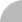簡易查詢 | 進階查詢 | 熱門排行 | 我的研究室